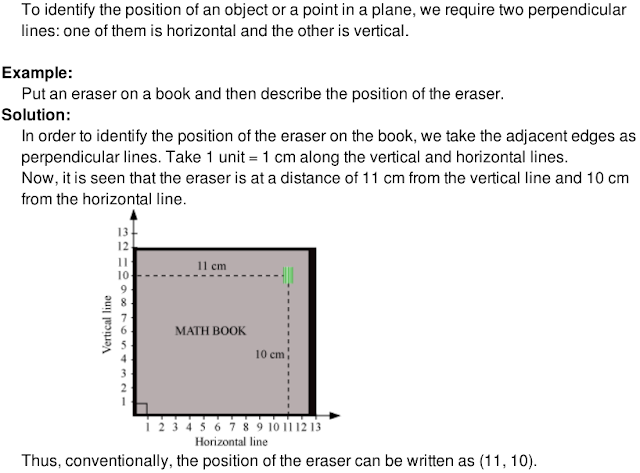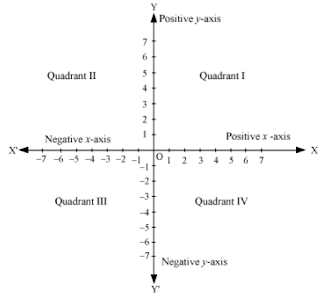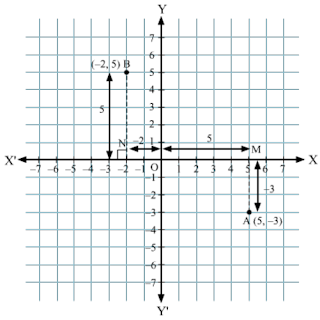## Revision Notes of Ch 3 Coordinate Geometry Class 9th Math

Topics in the Chapter

• Cartesian plane and the terms associated with it
• Cartesian system
• Coordinate Geometry
• Relationship between the signs of the coordinates of a point and the quadrant of the point in which it lies.
• Location of the point in the plane when its coordinates are given
• Plotting a point in the Cartesian Plane

Cartesian plane and the terms associated with itCartesian system

A Cartesian system consists of two perpendicular lines: one of them is horizontal and the other is vertical.

The horizontal line is called the x- axis and the vertical line is called the y -axis. The point of intersection of the two lines is called origin, and is denoted by O.• XOX' is called the x-axis; YOY' is called the y-axis; the point O is called the origin,

• Positive numbers lie on the directions of OX and OY.

• Negative numbers lie on the directions of OX' and OY'.

• OX and OY are respectively called positive x-axis and positive y-axis.

• OX' and OY are respectively called negative x-axis and negative y-axis.

• The  axes divide the plane into four equal pans. The four parts are called quadrants,  numbered l, II, Ill and IV, in anticlockwise from positive x-axis, OX,

• The plane is also called co-ordinate plane or Cartesian plane or xy-plane.

Coordinate Geometry
Example:
Name the quadrant or the axis in which the points (5, –4), (2, 7) and (0, –9) lie?

• The coordinates of the point (5, –4) are of the form (+, –). Hence, (5, –4) lie in quadrant IV

• The coordinates of the point (2, 7) are of the form (+, +). (2, 7) lie in quadrant I.

• The coordinates of the point (0, –9) are of the form (0, b). (0, –9) lie on the y-axis

• The coordinates of a point on the coordinate plane can be determined by the following conventions.

• The x-coordinate of a point is its perpendicular distance from the y-axis, measured along the x-axis (positive along the positive x-axis and negative along the negative x-axis). The x-coordinate is also called the abscissa.

• The y-coordinate of a point is its perpendicular distance from the x-axis, measured along the y-axis ( positive along the positive y-axis and negative along the negative y -axis). The y-coordinate is also called the ordinate.

• In stating the coordinates of a point in the coordinate plane, the x-coordinate comes first
and then the y-coordinate. The coordinates are placed in brackets.It is observed that
x-coordinate of point A is 5
y-coordinate of point A is 2
Coordinates of point A are (5, 2).
x-coordinate of point C is –5
y-coordinate of point C is 2
Coordinates of point C are (–5, 2).

Note: The coordinates of the origin are (0, 0). Since the origin has zero distance from both
the axes, its abscissa and ordinate are both zero.

Relationship between the signs of the coordinates of a point and the quadrant of the point in which it lies

• The 1st quadrant is enclosed by the positive x-axis and positive y-axis. So, a point in the 1st quadrant is in the form (+, +).

• The 2nd quadrant is enclosed by the negative x-axis and positive y-axis. So, a point in the 2nd quadrant is in the form (–, +).

• The 3rd quadrant is enclosed by the negative x-axis and the negative y-axis. So, the point in the 3rd quadrant is in the form (–, –).

• The 4th quadrant is enclosed by the positive x-axis and the negative y-axis. So, the point in the 4th quadrant is in the form (+, –).Note: The coordinates of the point on the x-axis are of the form (a, 0) and the coordinates
of the point on the y-axis are of the form (0, b), where a, b are real numbers.

Plotting a point in the Cartesian Plane

We can plot a point in the Cartesian plane, if the coordinates of the points are given.

Example: Plot the points A (5, –3) and B (–2, 5) on the Cartesian plane.

To plot A (5, –3):

(1) Move 5 units along OX and mark the endpoint as M.
(2) From M and perpendicular to the x-axis, move 3 units along OY'. Mark the endpoint as A.
This is the location of the point (5, –3) on the Cartesian plane.

To plot B (–2, 5):

(1) Move 2 units along OX' and mark the endpoint as N.
(2) From N and perpendicular to the x-axis, move 5 units along OY. Mark the endpoint as B.
This is the location of the point (–2, 5) on the Cartesian plane.

• Points A and B are plotted in the following graph.